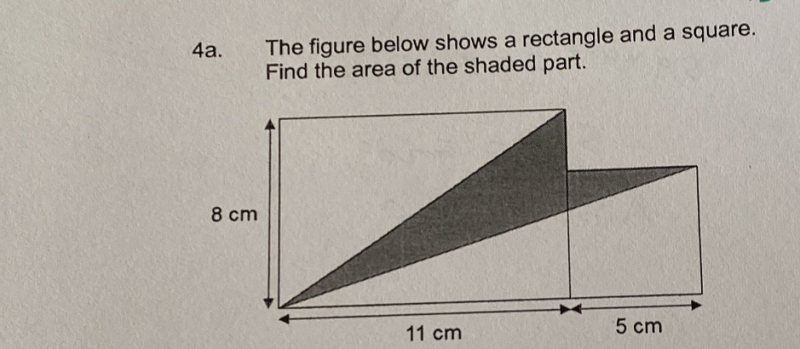# QuestionArea of square = 5 x 5 = 25

Area of triangle that is half the rectangle = (11 x 8)/2 = 44

Area of triangle unshaded on bottom-right = (1/2)(11+5) x 5 = 40

Therefore, area of shaded part = 25+44-40 = 29 cm2